MATH EXPLORERS' CLUB Cornell Department of Mathematics

Ehrenfeucht-Fraïssé games

 Introduction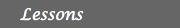1. History of EF games 2. Description 3. Examples 4. FO logic for graphs 5. EF games & FO logic 6. Applications ReferencesFirst order logic (FO)

We will only give a brief introduction to first order logic, adapted to the language of directed and undirected graphs. In the language of graphs we denote by E(x,y) the edge relation between two nodes x and y. We say that E(x,y) is true on the graph A, if there is an edge from node x to node y. Otherwise, if there is no edge from x to y, we say E(x,y) is false.

Let us look at the directed graph B depicted below: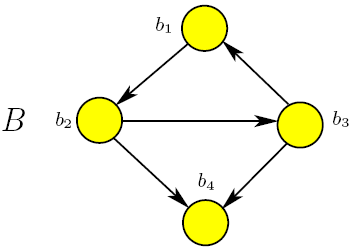• E(1,2), E(2,3), E(2,4), E(3,1), E(3,4) are true on graph B.
• E(1,3), E(1,4), E(2,1), E(2,4), E(3,2), E(4,1), E(4,2), E(4,3) are false on graph B.
Let us modify the graph B so that it is now undirected: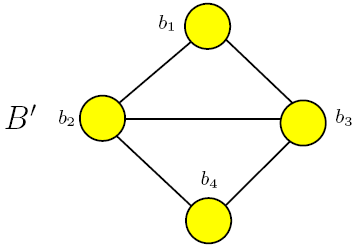• E(1,2), E(2,1), E(2,3), E(3,2), E(2,4), E(4,2), E(3,1), E(1,3), E(3,4), E(4,3) are now true on B'.
• E(1,4), E(4,1) are false on the graph B'.

E(1,2) is an example of an atomic formula in the language of graphs. Starting from atomic formulas, we can make propositions. Namely we take conjuctions (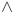), disjunctions (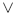) and negations of atomic formulas (¬). Negation has priority over conjuctions and disjunctions. Beyond this, the paranthesis dictate the order in which to proceed.

For instance, the proposition P = E(1,2)¬ E(1,3) is true on the graph B, and false on the graph B'.
In practice, in A and B are two propositions, the following rules, called de Moivre's laws are useful: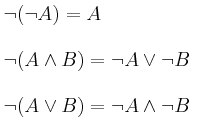First order logic (in short FO) is richer then propositional logic. Its lexicon contains not only the symbols,, ¬, from propositional logic, but also the symbols (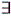) for "there exists" and (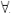) representing "forall", along with various symbols to represent variables, constants, functions and relations.

We use lower case letters from the end of the alphabet to denote variables. For example x,y are variables, 1,2 constants, E(x,y) the edge relation, E(1,2) a proposition, E(1,y) is again a relation, which evaluates to true on those nodes y for which there exists an edge between 1 and y.

Example: Take the FO sentence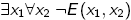.
This can be translated into the following question "Does there exist a node x1, such that forall nodes x2, there does not exist an edge from x1 to x2?". Or, simply asked, "Does there exist a node which does not have an edge to any other node?".
• In graph B, we can find such a node, it is b4, so we say that the given FO sentence is true on B.
• In graph B', we cannot find a node with this property, as every node in B' has at least 2 edges connecting it to its neighbors, so the FO sentenceis false on B'.
One concept that is useful to the discussion from the following chpaters is the quantifier rank of a FO formula.
Definition: Let f be a first order formula. The quantifier rank of f, denoted by qr(f) is the depth of the quantifier nesting in f. More formally, qr(f) is defined inductively as follows:
• If f is atomic, then qr(f) = 0
• If f is of the form ¬g, for some FO formula g, then qr(f) = qr(g)
• If f is of the form gh, or gh, then qr(f) = max {qr(g), qr(h)}
• If f is of the formx g, orx g, then qr(f) = qr(g) + 1
Example:
• the quantifier rank of E(x,y) is 0, as E is quantifier free. We write qr(E(x,y)) = 0.
• qr() = 2
Let us conclude our brief introduction to first order logic explained in the language of graphs by the proposing to the reader the following exercise:
Exercise 1: Consider the FO sentence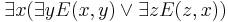1. Explain what the meaning of the formula is.
2. Decide whether it is true when considered over the graph B.
3. What is its quantifier rank?

 Top Next lesson